Mesh Sneaker Casual
Rated 3.5/5 based on 11 customer reviews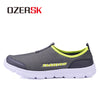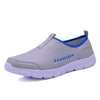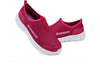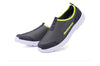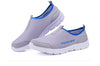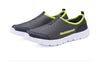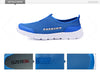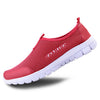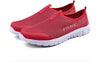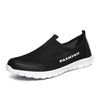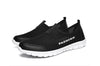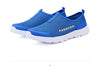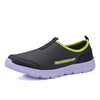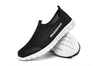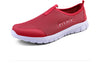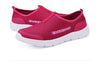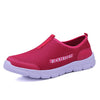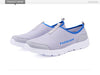# Mesh Sneaker Casual

• Product SKU: 21189592-dark-gray-6
• Availability: In Stock
\$75.72 \$36.72
Hurry! only 0 left in stock!
Color
• Order within
Your message has been successfully sent to the store owner!
Shipping
PS: Please choose according to the length of the foot, please do not use the length of the insole to choose.
Size 4.5=34   fit for foot length is 22cm.
Size 5=35      fit for foot length is 22.5cm.
Size 5.5=36   fit for foot length is 23cm.
Size 6=37      fit for foot length is 23.5cm.
Size 6.5=38   fit for foot length is 24cm.
Size 7=39      fit for foot length is 24.5cm.
Size 7.5=40   fit for foot length is 25cm.
Size 8=41      fit for foot length is 25.5cm.
Size 8.5=42   fit for foot length is 26cm.
Size 9.5=43   fit for foot length is 26.5cm.
Size 10=44    fit for foot length is 27cm.
Size 11=45    fit for foot length is 27.5cm.
Size 12=46    fit for foot length is 28cm.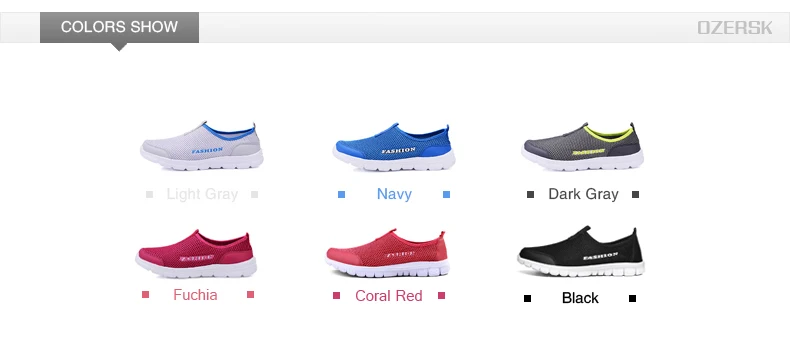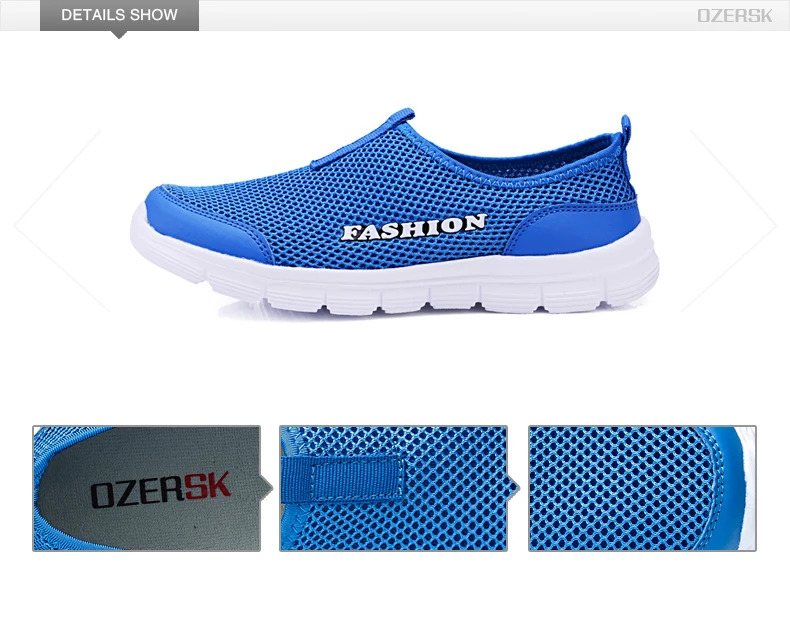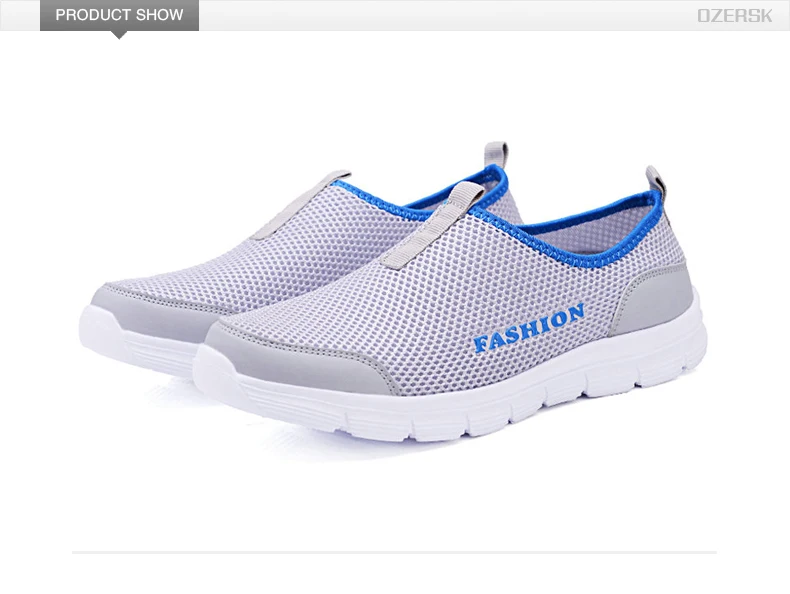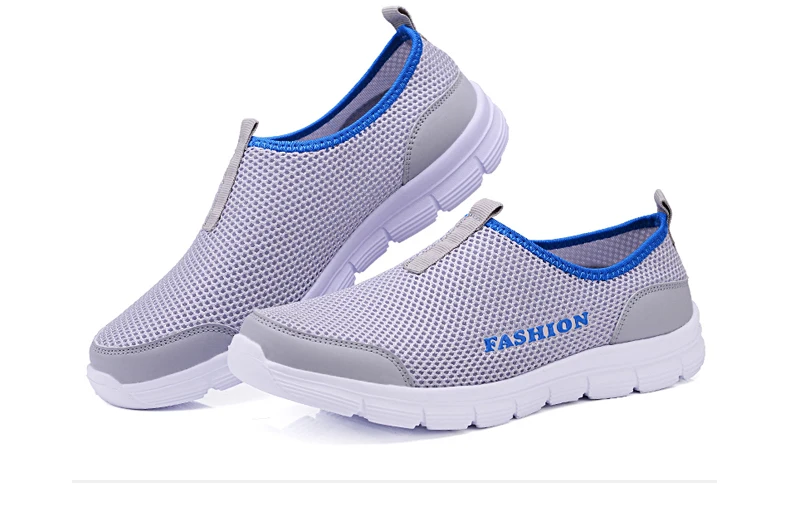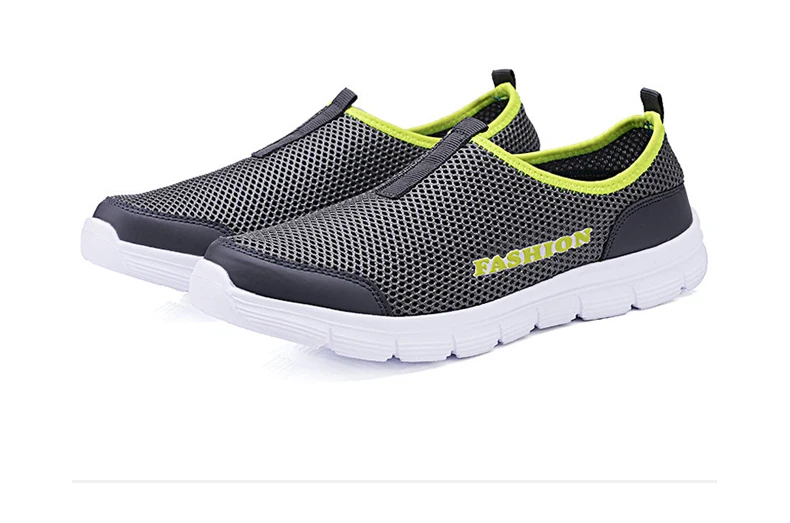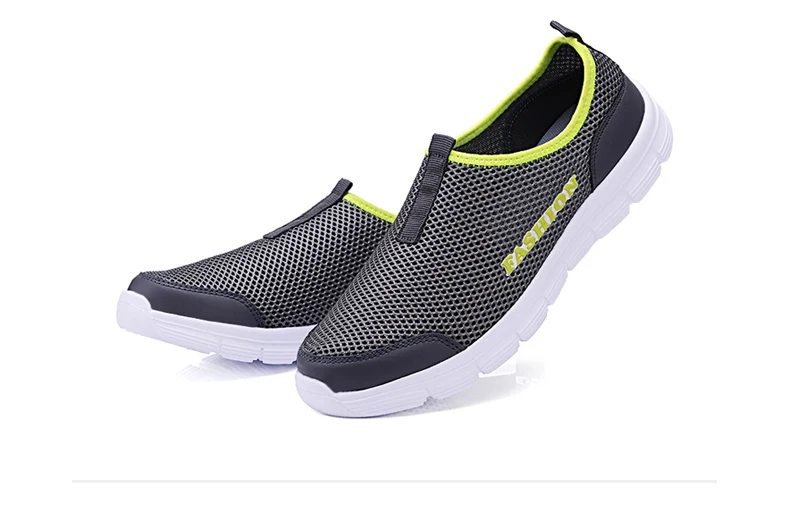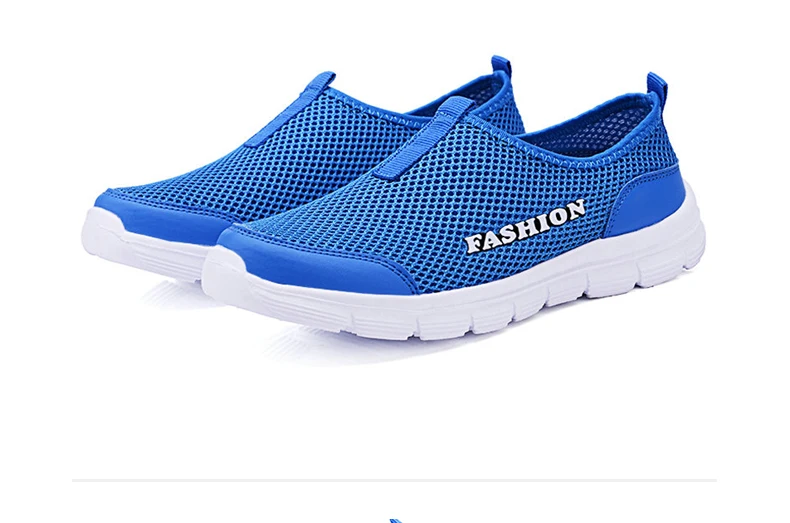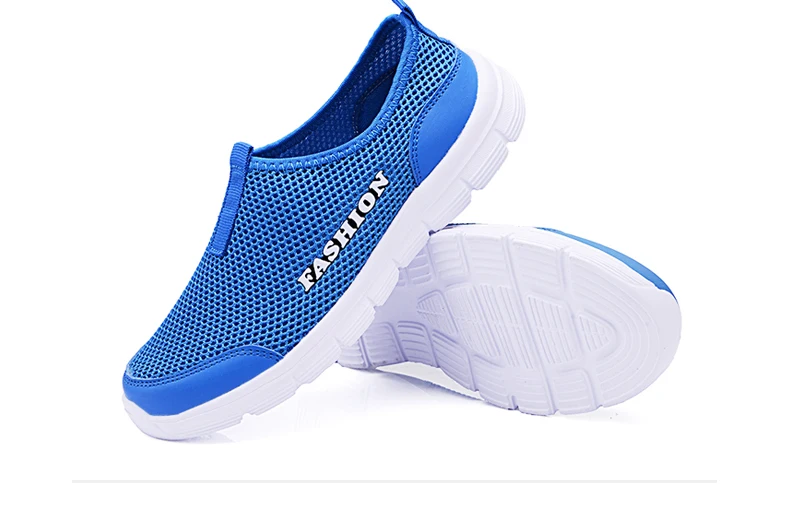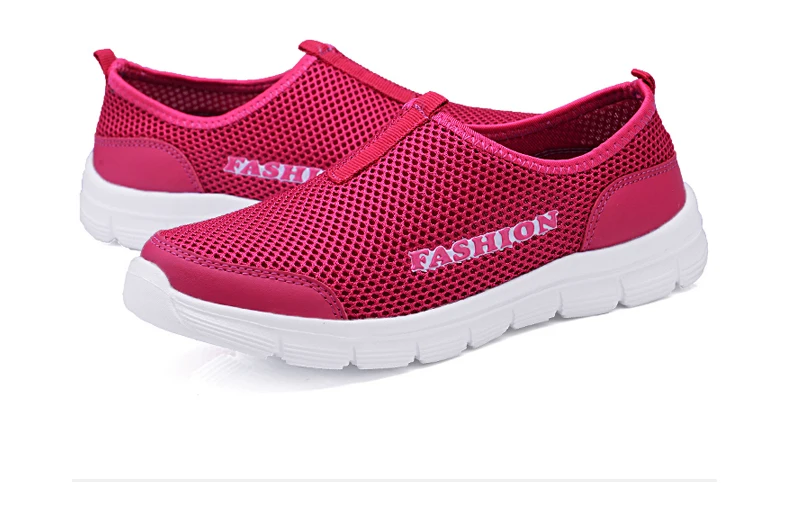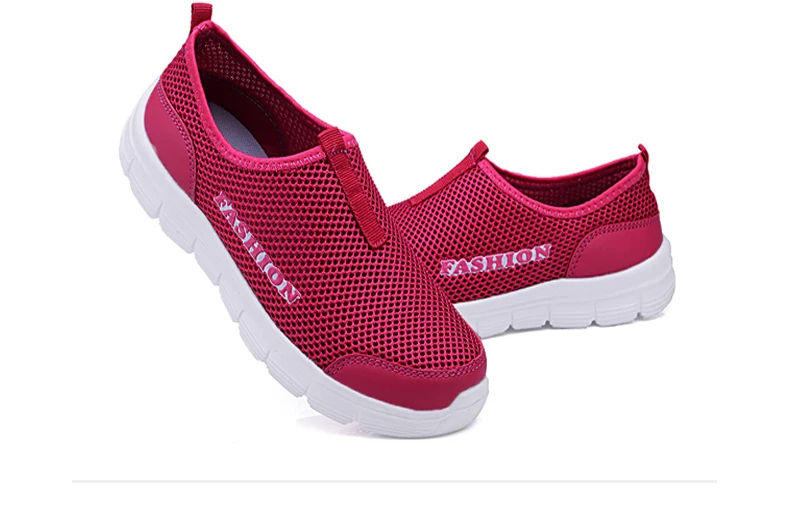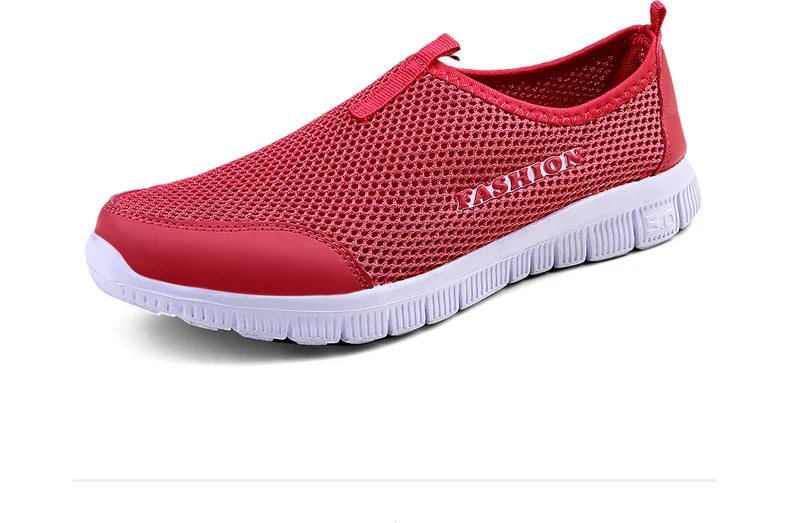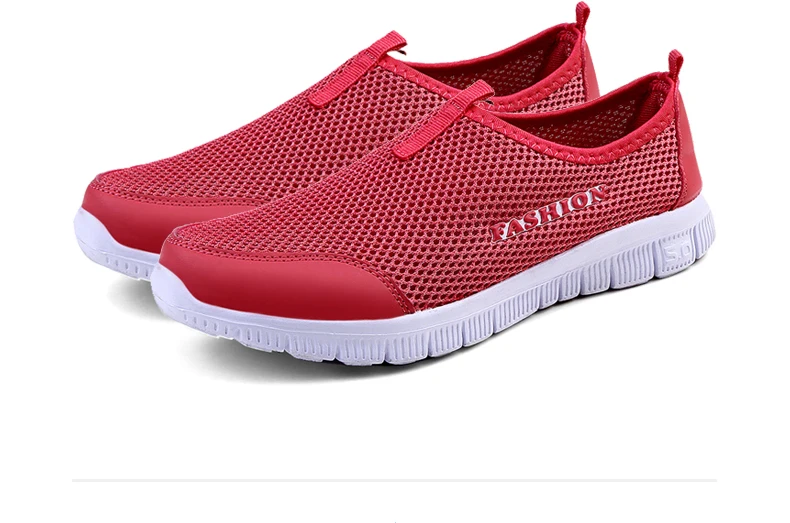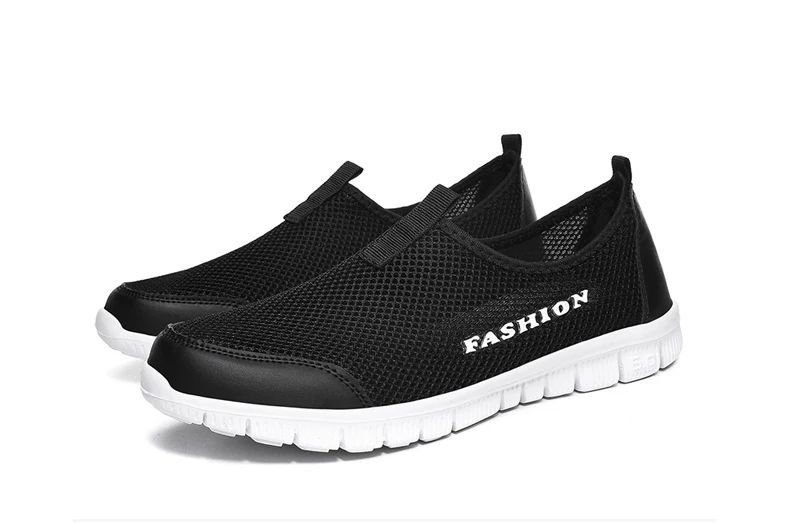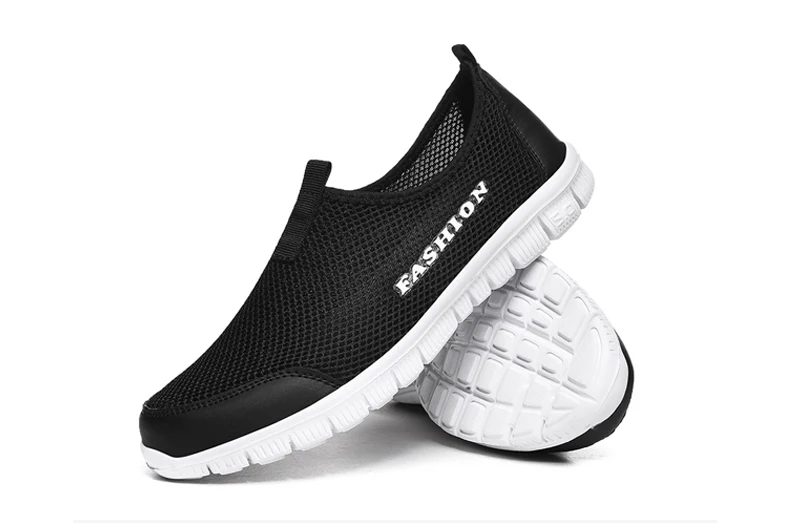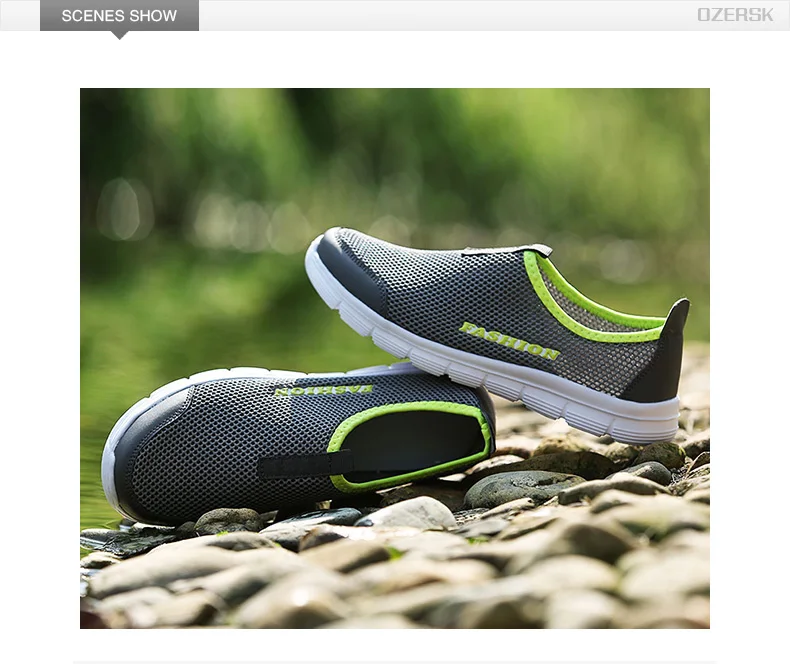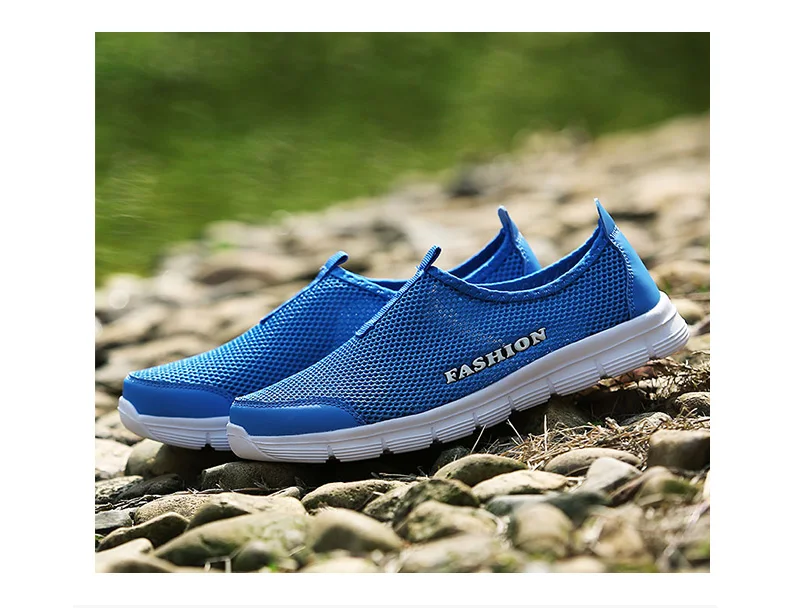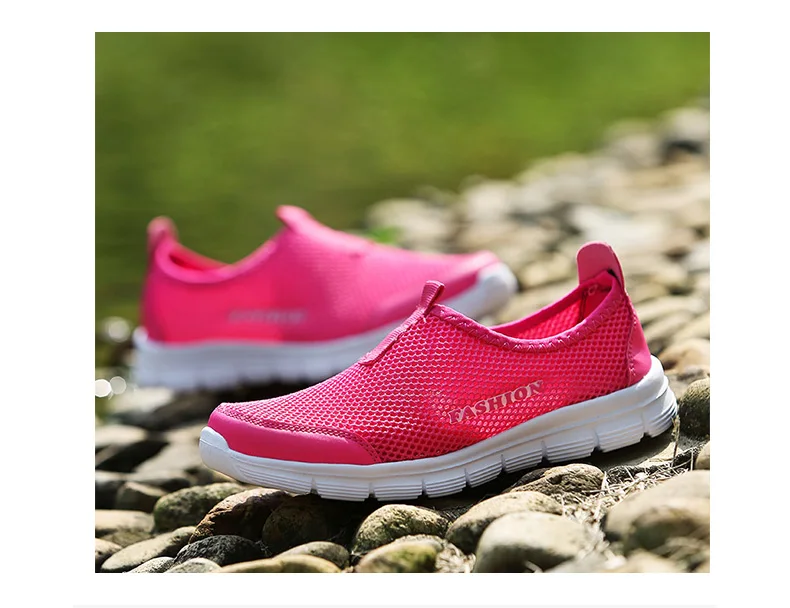Shipping PS: Please choose according to the length of the foot, please do not use the length of the insole to choose. Size 4.5=34   fit for foot length is 22cm.     Size 5=35      fit for foot length is 22.5cm.  Size 5.5=36   fit for foot length is 23cm.     Size 6=37      fit for foot length is 23.5cm.  Size 6.5=38   fit for foot length is 24cm.     Size 7=39      fit for foot length is 24.5cm.    Size 7.5=40   fit for foot length is 25cm.       Size 8=41      fit for foot length is 25.5cm.  Size 8.5=42   fit for foot length is 26cm.     Size 9.5=43   fit for foot length is 26.5cm.  Size 10=44    fit for foot length is 27cm.     Size 11=45    fit for foot length is 27.5cm.  Size 12=46    fit for foot length is 28cm.